# How Light's Speed was Determined

This is part of the HSC Physics course under the topic Electromagnetic Spectrum.

### HSC Physics Syllabus

• Conduct investigations of historical and contemporary methods used to determine the speed of light and its current relationship to the measurement of time and distance. (ACSPH082)

## Historical Methods to Measure Light's Speed

### 1675 – Ole Rømer 200,000 km/sec

Ole Rømer was a Danish astronomer who was observing the orbital motion of Io (one of the four satellites of Jupiter), when he determined the speed of light. The orbital period of Io around Jupiter is about 1.7 Earth days, and during each orbit, Io is eclipsed by Jupiter once as viewed from Earth.

However, after multiple viewings, Rømer realised the time between each eclipse varies with the relative motion of Earth to Jupiter. Rømer measured that the difference in the longest observed orbital period of Io and the shortest period was 22 minutes. He explained that this was due to the finite speed of light.Diagram source: Shea, J.H., 1998. Ole Rømer, the speed of light, the apparent period of Io, the Doppler effect, and the dynamics of Earth and Jupiter. American Journal of Physics 66, 561–569 .

Unlike incorrectly stated by numerous analyses of Rømer's work, the deviation of Io's orbital period does not depend on the distance between Earth and Jupiter. In fact, the changing observed orbital period is due to Earth's relative velocity.

Suppose the start of Io's orbit was recorded at point L, and by the time Io completes one revolution, Earth is at point K. Light would require additional time to travel the distance between point L and K, resulting in a longer apparent orbital period for Io. If Earth did not move at remained at point L, then there would be no change in the observed orbital period.

Conversely, if the start of Io's orbit was recorded at point F, and by the time Io completes one revolution, Earth is at point G, light would require less time to reach Earth. This results in a shorter apparent orbital period for Io.Figure: True orbital period is observed when Earth's relative velocity to Jupiter is zero.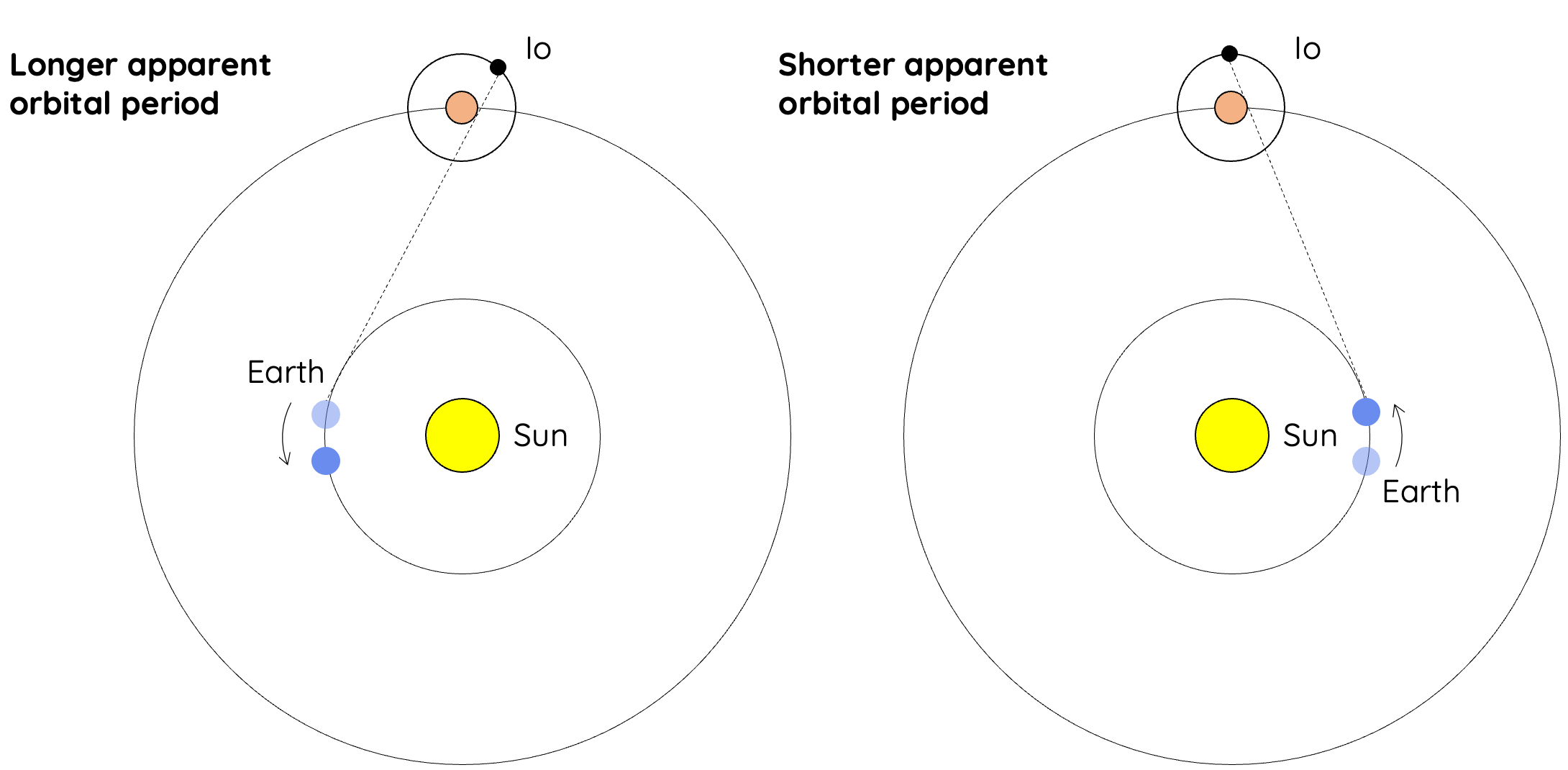Figure: Left: Longest apparent orbital period is observed when Earth's receding velocity (moving away) relative to Jupiter is the greatest. Right: Shortest apparent orbital period of Io is observed when Earth's relative velocity towards Jupiter is the greatest.

Due to the poor methods for timekeeping, Rømer was unable to accurately measure the time deviation between successive orbits of Io as this was on a scale of a few seconds. However, he measured the difference in period of multiple orbits at once and found that the largest total difference observed was 22 minutes. In other words, the time deviation of each successive orbit of Io accumulated to a maximum of 22 minutes. This difference in time corresponded to the time taken by light to traverse the diameter of Earth's orbit around the Sun. As such, an estimation of light’s speed was made by dividing the diameter of Earth’s orbit by 22 minutes.

Rømer's estimation of light's speed was inaccurate due to several reasons. Earth's orbital diameter was not accurately known; the estimation in total time difference was in fact shorter than 22 minutes. Although Rømer's work was not accurate, it was very impactful as it showed the speed of light was finite.

### 1728 – James Bradley 301,000 km/sec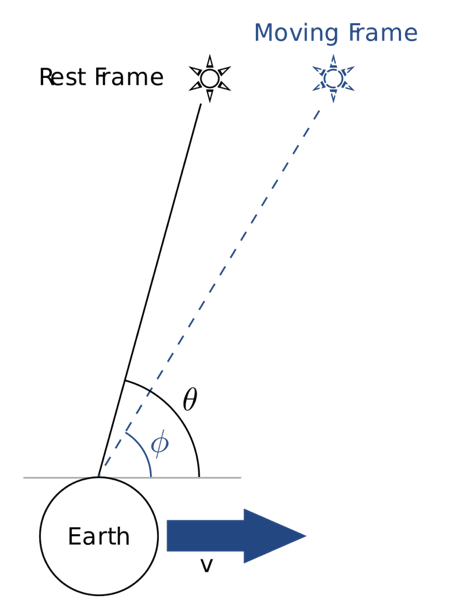Figure: Rest frame refers to the true position of a star while moving frame refers to the displaced perceived position of a star due to the relative velocity of Earth. The latter observation is called stellar aberration.

Bradley used stellar aberration to calculate the relative speed of light. Stellar aberration refers to the astronomical phenomenon of when stars appear to be moving about their true position. This causes them to seem displaced when in fact their position has not changed. Bradley used the angle of displacement measured from Earth as well as the relative velocity of Earth to calculate the speed of light. The angle of aberration is related to the ratio between Earth (observer)’s relative speed to the speed of light.

### 1849 – Hippolyte Louis Fizeau 313,300 km/sec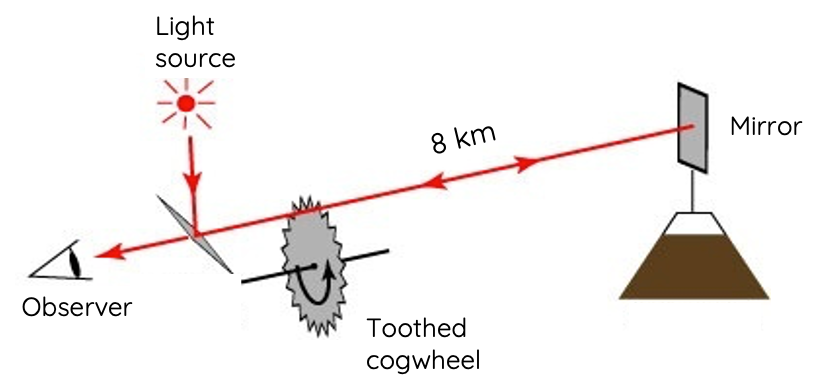Fizeau used optics and carefully a manipulated toothed wheel to investigate the speed of light. A reflective mirror was placed far away from the source of light. Upon reaching the mirror, the light would return back to the observer standing next to the source of light.

Depending on the angular speed of the wheel, the reflected light may or may not pass through the same gap between the teeth on the spinning wheel. Fizeau adjusted the angular speed of the toothed wheel so that the returning light ray  and along with the known distance between the wheel and the mirror, he was able to calculate the speed of light to be 313,300 km/sec.

### 1862 – Leon Foucault 298,000 km/sec

Foucault’s experiment exploited the same principle as Fizeau, but he utilised rotating mirrors instead of a toothed wheel.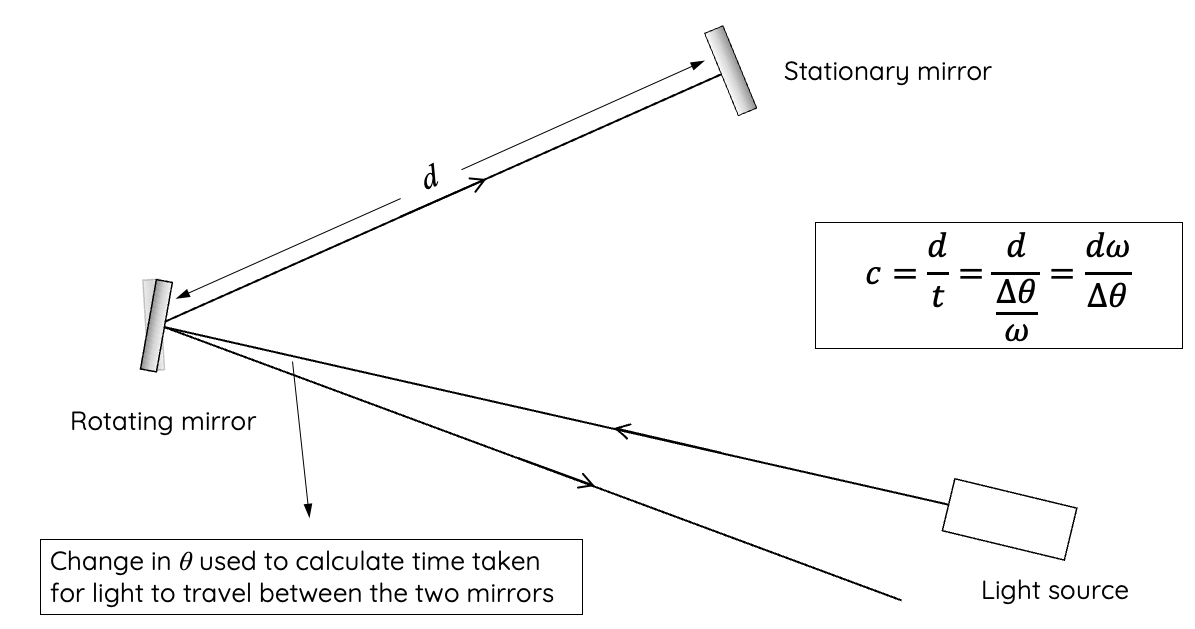A beam of light was shone onto a distant rotating mirror which reflected the light onto a nearby fixed mirror. This fixed mirror then directed the light back to the first rotating mirror. Since the first mirror was constantly rotating, the angle at which the light first reached the rotating mirror would be different to the angle the light makes when it returns from the fixed mirror.

Foucault was able to use the angle displaced by the mirror and its angular speed to calculate the time of flight for light. Using the time of flight and the distance between the rotating and fixed mirrors, Foucault calculated light's speed to be 298 000 000 m/sec.

Foucault repeated the experiment by passing the light source through a body of water. He found that the speed of light was slower in water than in air.

## Contemporary Methods to Measure Light's Speed

### 1907 – Rosa & Dorsey 299,788 km/sec

The scientific breakthrough in electromagnetism by James Maxwell in 1860s shed light on a new way of measuring speed of light. Rosa and Dorsey used the electric permittivity (ε) and magnetic permeability (µ) constants to calculate the speed of light.

$$c=\frac{1}{\sqrt{εμ}}$$

This method differed from historical methods in that it did not require an measurement of light's time of flight.

### 1958 – Froome 299,972,500 m/s

Froome’s methodology could not have been possible without Maxwell’s contribution to electromagnetic waves. Froome studied the interference pattern of radiowaves to obtain the speed of light. See below for a detailed explanation of interferometry using visible light (works the same way for radiowaves)

### 1973 – Evenson et al 299,972,457 m/s

Evenson et al utilised lasers with high spectral stability to measure the speed of light. Like Froome, this experiment also involved interferometry to measure the wavelength of light.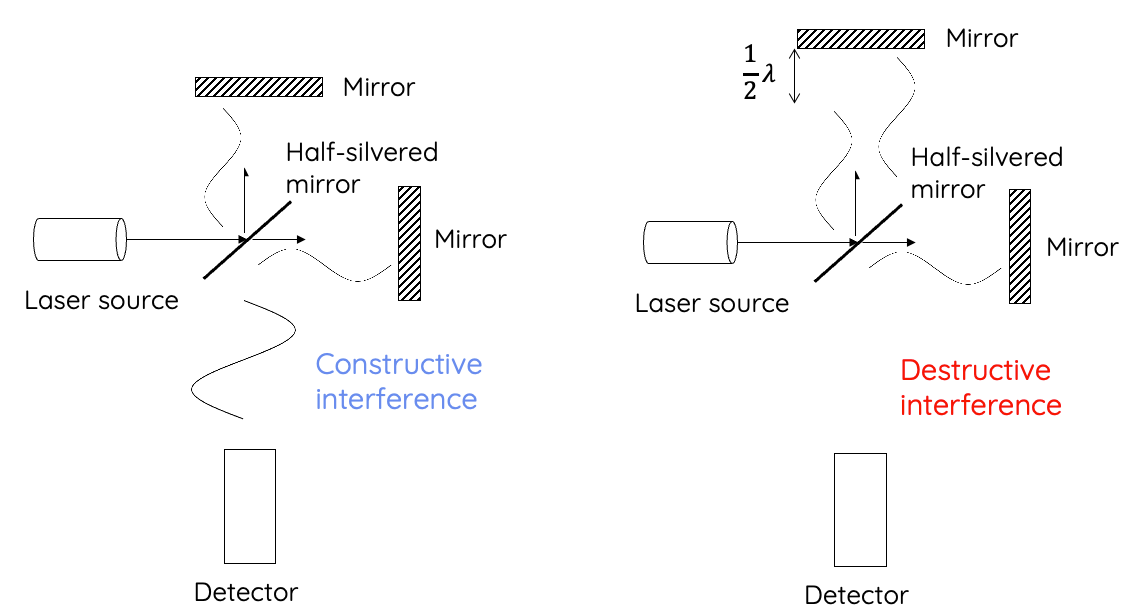Figure: simplified experimental set-up for interferometry used to measure light's speed.

In this method, a laser source is fired at a half-silvered mirror which splits the light ray into two perpendicular rays that travel to two stationary mirrors and back. The two stationary mirrors are equidistant from the half-silvered mirror, so the two light rays will take the same amount of time to return. When they meet in the middle, they will undergo constructive interference, resulting in a new wave that has twice the amplitude.

Now, the same experiment is repeated but this time one of the stationary mirrors is moved back so the distance between it and the half-silvered mirror is increased. When the mirror is moved back by exactly half a wavelength of the light wave, it will cause the two waves to undergo destructive interference, so there will be no waves detected.

In laser interferometry, a laser source with known frequency is used, and the wavelength of the laser is measured by determining the distance by which the stationary mirror needs to be moved to cause destructive interference. Light’s speed is then calculated by multiplying the frequency and wavelength.

## Light’s Relationship with Time and Distance

• In the modern age, due to the constancy of light’s speed, standard distance and time units are defined using speed of light.
• The concept of distance and time is not constant as they change with frames of reference. This is due to Albert Einstein's theory of Special Relativity and his postulate - speed of light is constant in a vacuum, regardless of the observer. This will be covered in more detail later in the module.

Constancy of light has two effects:

• Time becomes dilated (each second becomes longer) when an observer is at relativistic motion with the observed frame of reference.
• Length becomes shorter when an observer is travelling at relativistic motion.

As a result, distance (metre) and time (second) are measured and defined in terms of speed of light, which is a constant value.

• The definition of a metre is the distance light travels in 1/299,792,458 second.
• The definition of a second is the time light takes to travel 299,792,458 metres.

Previous section: Maxwell's Contribution to Electromagnetism

Next section: Introduction to Spectroscopy